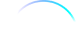## FANDOM

49,472 Pages

"And the square of the hypotenuse of a right triangle..."
"...is equal to the sum of the square of the other two. Two something. Which I once heard, but never understood."

The Pythagorean theorem states that in a right triangle, the length of the hypotenuse squared is equal to the sum of the square of the lengths of the other two sides, and was a very important equation in the study of geometry. It was often represented by the following equation:

$a^2+b^2=c^2$

where a and b are each the legs of the triangle and c is the hypotenuse.

Commander William T. Riker used the theorem to trick the android Lore in 2364. He stated the first part of the theorem and Lore completed it without thinking, revealing that he knew more than he was letting the USS Enterprise-D crew think. (TNG: "Datalore")

Community content is available under CC-BY-NC unless otherwise noted.